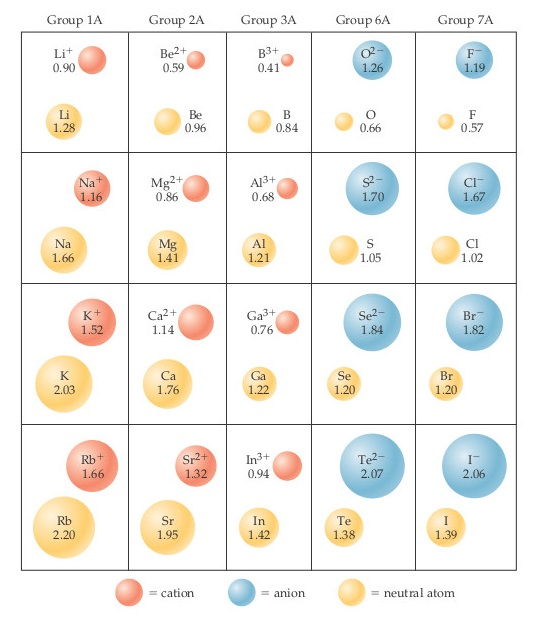# Problem: In the ionic compounds LiF, NaCl, KBr, and RbI, the measured cation-anion distances are 2.01 Å (Li–F), 2.82 Å (Na–Cl), 3.30 Å (K–Br), and 3.67 Å (Rb–I), respectively.What estimates of the cation–anion distance would you obtain for these four compounds using neutral atom bonding atomic radii? Are these estimates as accurate as the estimates using ionic radii?

⚠️Our tutors found the solution shown to be helpful for the problem you're searching for. We don't have the exact solution yet.

###### Problem Details
In the ionic compounds LiF, NaCl, KBr, and RbI, the measured cation-anion distances are 2.01 Å (Li–F), 2.82 Å (Na–Cl), 3.30 Å (K–Br), and 3.67 Å (Rb–I), respectively.What estimates of the cation–anion distance would you obtain for these four compounds using neutral atom bonding atomic radii? Are these estimates as accurate as the estimates using ionic radii?